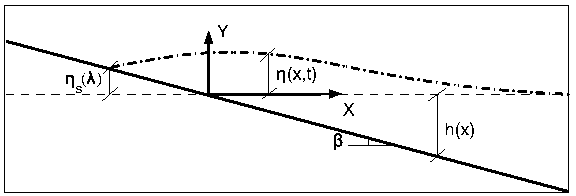The nonlinear evolution of a wave over a sloping beach is theoretically and numerically challenging due to the moving boundary singularity. Yet, it is important to have a good estimate of the shoreline velocity and associated runup-rundown motion, since they are crucial for the planning of coastal flooding and of coastal structures. As explained in the previous section, Synolakis (1987) solved this problem as a boundary value problem considering canonical bathymetry. Kânoglu (2004) solved nonlinear evolution of any given wave form over a sloping beach as an initial value problem (Fig. 1). It is proposed that any initial waveform can first be represented in the transform space using the linearized form of the Carrier-Greenspan transformation for the spatial variable, then the nonlinear evolutions of these initial waveforms can be directly evaluated. Later, Kânoglu and Synolakis (2006) solved the similar problem considering a more general initial condition, i.e., initial wave with velocity.

Kânoglu (2004) considers NSW equations () with slightly different nondimensionalization than (), i.e., using the reference length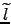as a scaling parameter, the dimensionless variables are introduced as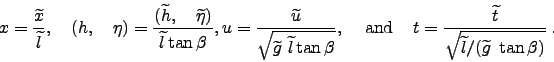(1)

Using the original Carrier-Greenspan transformation--without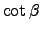coefficient in () and ()--it is possible to reduce the NSW equations to the following single second-order linear equation the same as ():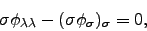(2)

using the Riemann invariants of the hyperbolic system (Carrier and Greenspan, 1958). The Carrier-Greenspan transformation not only reduces the nonlinear shallow water-wave equations into a second-order linear equation, but also fixes the instantaneous shoreline to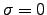in the-space as explained previously. Furthermore, a bounded solution at the shoreline combined with a given initial condition in terms of a wave profile at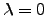in the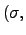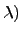-space,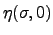implies the following solution in the transform space,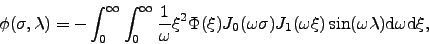(3)

where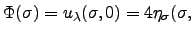. Further, given the initial waveform, the evolution of the water-surface elevation is now given by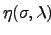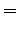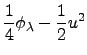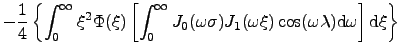(4)

where, again,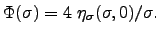Since it is important for coastal planning, simple expressions for shoreline runup-rundown motion and velocity are useful. Considering the shoreline corresponds toin the-space, (4) reduces to the following equation for the runup-rundown motion: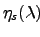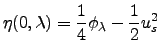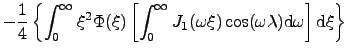(5)

Here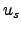and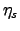represent shoreline velocity and motion, respectively. The singularity of the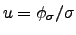at the shoreline () is removed with the consideration of the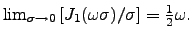The difficulty of deriving an initial condition in the-space is overcome by simply using the linearized form of the hodograph transformation for a spatial variable in the definition of initial condition. It is proposed that any initial waveform can first be represented in the transform space using the linearized form of the Carrier-Greenspan transformation for the spatial variable (() withoutcoefficient), then the nonlinear evolutions of these initial waveforms can be directly evaluated. Once an initial value problem is specified in the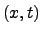-space as, the linearized hodograph transformation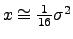is used directly to define the initial waveform in the-space,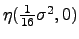. Thus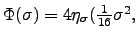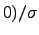is found, and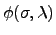follows directly through a simple integration, as in (4). Then it becomes possible to investigate any realistic initial waveform such as Gaussian and N-wave shapes employed in Carrier et al. (2003) and the isosceles and general N-waves defined by Tadepalli and Synolakis (1994). Again, solution in the physical space can be found using the Newton-Raphson algorithm proposed by Synolakis (1987) and later used by Kânoglu (2004), as presented in (A24a, b).

The shoreline runup-rundown motion and velocity are presented for one of the initial profiles given by Carrier et al. (2003):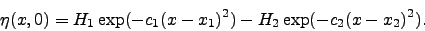(6)

The following initial profile can be obtained in the transform space after using the linearized form of the transformation for the spatial variable: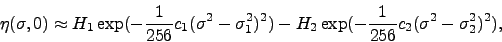(7)

which leads to the definition of the initial condition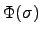: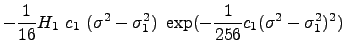(8)

Figure 2a compares the initial waveforms defined in the physical space as in (6) with the one resulting from the approximation of it, i.e., (calculated through (4)). The linearized form of the spatial variable in the definition of the initial waveforms gives satisfactory comparison. Figures 2b and 2c present the shoreline runup-rundown motion and velocity, respectively, calculated from equation (5) using the corresponding parts. It should be added that the solution presented here cannot be evaluated when the Jacobian of the transformation,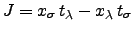, breaks down. Even though the transformation might become singular at certain points, the solution can still be obtained at other points, since local integration can be performed without prior knowledge of the dependent variables, unlike in numerical methods. This feature is discussed in detail in Synolakis (1987) and Carrier et al. (2003), and is not explained further in here.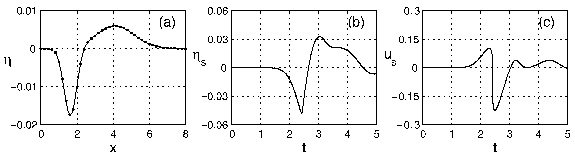References:

Carrier, G.F., T.T. Wu, and H. Yeh (2003): Tsunami runup and drawdown on a sloping beach. J. Fluid Mech., 475, 79-99.

Kânoglu, U. (2004): Nonlinear evolution and runup-rundown of long waves over a sloping beach. J. Fluid Mech., 513, 363-372.

Kânoglu, U., and C. Synolakis (2006): Initial value problem solution of nonlinear shallow water-wave equations. Phys. Rev. Lett., 97, 148501-148504.